##### Geometry For DummiesComplementary angles are two angles that add up to 90°, or a right angle; two supplementary angles add up to 180°, or a straight angle. These angles aren’t the most exciting things in geometry, but you have to be able to spot them in a diagram and know how to use the related theorems in proofs.

You use the theorems listed here for complementary angles:

• Complements of the same angle are congruent. If two angles are each complementary to a third angle, then they’re congruent to each other. (Note that this theorem involves three total angles.)

• Complements of congruent angles are congruent. If two angles are complementary to two other congruent angles, then they’re congruent. (This theorem involves four total angles.)

The following examples show how incredibly simple the logic of these two theorems is.

Complements of the Same Angle

Given: Diagram as shown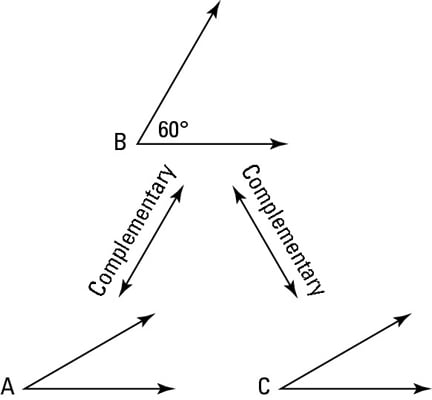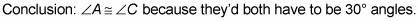Complements of Congruent Angles

Given: Diagram as shown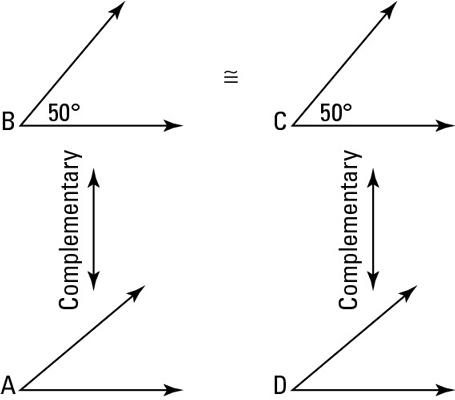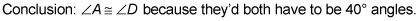Note: The logic shown in these two figures works the same when you don’t know the size of the given angles

And here are the two theorems about supplementary angles that work exactly the same way as the two complementary angle theorems:

• *Supplements of the same angle are congruent. If two angles are each supplementary to a third angle, then they’re congruent to each other. (This is the three-angle version.)

• *Supplements of congruent angles are congruent. If two angles are supplementary to two other congruent angles, then they’re congruent. (This is the four-angle version.)

The previous four theorems about complementary and supplementary angles come in pairs: One of the theorems involves three segments or angles, and the other, which is based on the same idea, involves four segments or angles. When doing a proof, note whether the relevant part of the proof diagram contains three or four segments or angles to determine whether to use the three- or four-object version of the appropriate theorem.

Take a look at one of the complementary-angle theorems and one of the supplementary-angle theorems in action: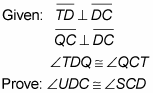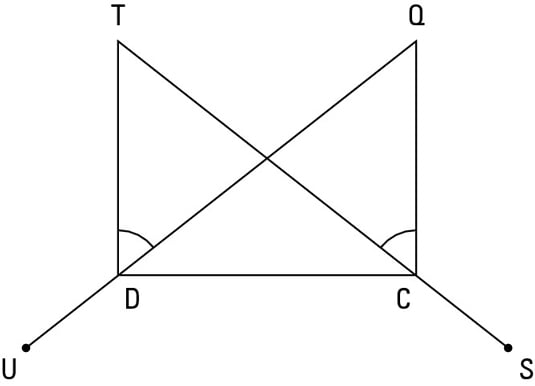Before trying to write out a formal, two-column proof, it’s often a good idea to think through a seat-of-the-pants argument about why the prove statement has to be true. Think of this argument as a game plan. Game plans are especially helpful for longer proofs, because without a plan, you might get lost in the middle of the proof.

When working through a game plan, you may find it helpful to make up arbitrary sizes for segments and angles in the proof. You can do this for segments and angles in the givens and, sometimes, for unmentioned segments and angles. You should not, however, make up sizes for things that you’re trying to show are congruent.

Game plan: In this proof, for example, you might say to yourself, “Let’s see. . . . Because of the given perpendicular segments, you have two right angles.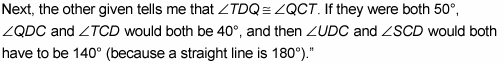That’s it.

Here’s the formal proof (each statement is followed by the reason).

Statement 1: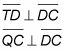Reason for statement 1: Given. (Why would they tell you this? See reason 2.)

Statement 2: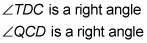Reason for statement 2: If segments are perpendicular, then they form right angles (definition of perpendicular).

Statement 3: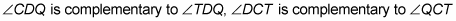Reason for statement 3: If two angles form a right triangle, then they’re complementary (definition of complementary angles).

Statement 4: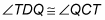Reason for statement 4: Given.

Statement 5: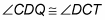Reason for statement 5: If two angles are complementary to two other congruent angles, then they’re congruent.

Statement 6: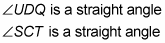Reason for statement 6: This is assumed from the diagram.

Statement 7: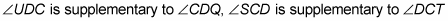Reason for statement 7: If two angles form a straight angle, then they’re supplementary (definition of supplementary angles).

Statement 8: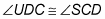Reason for statement 8: If two angles are supplementary to two other congruent angles, then they’re congruent.

Note: Depending on where your geometry teacher falls on the loose-to-rigorous scale, he or she might allow you to omit a step like step 6 in this proof because it’s so simple and obvious. Many teachers begin the first semester insisting that every little step be included, but then, as the semester progresses, they loosen up a bit and let you skip some of the simplest steps.